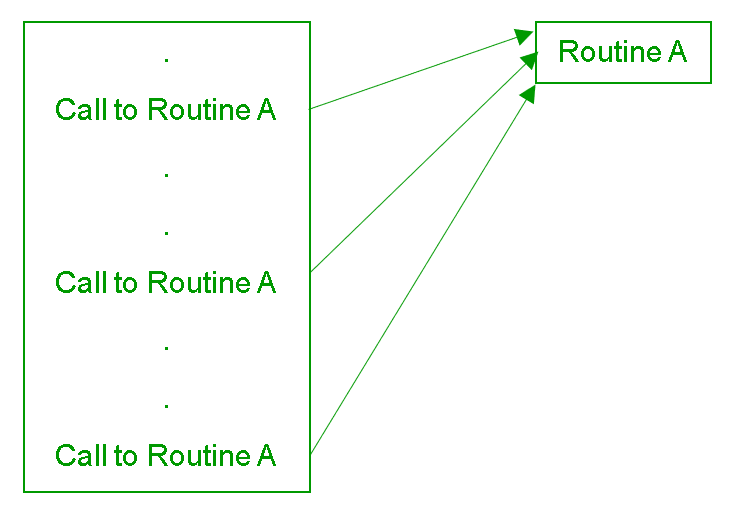# How to pass multiple arguments to function ?

• Difficulty Level : Expert
• Last Updated : 01 Feb, 2022

A Routine is a named group of instructions performing some tasks. A routine can always be invoked as well as called multiple times as required in a given program.When the routine stops, the execution immediately returns to the stage from which the routine was called. Such routines may be predefined in the programming language or designed or implemented by the programmer. A Function is the Python version of the routine in a program. Some functions are designed to return values, while others are designed for other purposes.
We pass arguments in a function, we can pass no arguments at all, single arguments or multiple arguments to a function and can call the function multiple times.
Example:

## Python

 `# no argument is passed` `# function definition``def` `displayMessage():``      ``print``(``"Geeks for Geeks"``)` `# function call``displayMessage()`

Output:

`Geeks for Geeks`

In the above program, the displayMessage() function is called without passing any arguments to it.

## Python

 `# single argument is passed` `# function definition``def` `displayMessage(msg):``       ``print``(``"Hello "``+``msg``+``" !"``)` `msg ``=` `"R2J"` `# function call``displayMessage(msg)`

Output:

`Hello R2J !`

In the above program, the displayMessage() function is called by passing an argument to it. A formal argument is an argument that is present in the function definition. An actual argument is an argument, which is present in the function call.
Passing multiple arguments to a function in Python:

• We can pass multiple arguments to a python function by predetermining the formal parameters in the function definition.

## Python

 `# multiple arguments are passed` `# function definition``def` `displayMessage(argument1, argument2, argument3):``          ``print``(argument1``+``" "``+``argument2``+``" "``+``argument3)` `# function call``displayMessage(``"Geeks"``, ``"4"``, ``"Geeks"``)`

• Output:
`Geeks 4 Geeks`
• In the above program, multiple arguments are passed to the displayMessage() function in which the number of arguments to be passed was fixed.
• We can pass multiple arguments to a python function without predetermining the formal parameters using the below syntax:
`def functionName(*argument)`
• The * symbol is used to pass a variable number of arguments to a function. Typically, this syntax is used to avoid the code failing when we don’t know how many arguments will be sent to the function.

## Python

 `# variable number of non keyword arguments passed` `# function definition``def` `calculateTotalSum(``*``arguments):``    ``totalSum ``=` `0``    ``for` `number ``in` `arguments:``        ``totalSum ``+``=` `number``    ``print``(totalSum)` `# function call``calculateTotalSum(``5``, ``4``, ``3``, ``2``, ``1``)`

• Output:
`15`
• In the above program, the variable number of arguments are passed to the displayMessage() function in which the number of arguments to be passed is not predetermined. (This syntax is only used to pass non-keyword arguments to the function.)
• We can pass multiple keyword arguments to a python function without predetermining the formal parameters using the below syntax:
`def functionName(**argument)`
• The ** symbol is used before an argument to pass a keyword argument dictionary to a function, this syntax used to successfully run the code when we don’t know how many keyword arguments will be sent to the function.

## Python

 `# variable number of keyword arguments passed` `# function definition``def` `displayArgument(``*``*``arguments):``    ``for` `arg ``in` `arguments.items():``        ``print``(arg)` `# function call``displayArgument(argument1 ``=``"Geeks"``, argument2 ``=` `4``,``                ``argument3 ``=``"Geeks"``)`

• Output:
```('argument2', 4)
('argument3', 'Geeks')
('argument1', 'Geeks')```
• In the above program, variable number of keyword arguments are passed to the displayArgument() function.

Here is a program to illustrate all the above cases to pass multiple arguments in a function.

## Python

 `# single argument, non keyword argument``# and keyword argument are passed` `# function definition``def` `displayArguments(argument1, ``*``argument2, ``*``*``argument3):``    ` `    ``# displaying predetermined argument``    ``print``(argument1)``    ` `    ``# displaying non keyword arguments``    ``for` `arg ``in` `argument2:``        ``print``(arg)``    ` `    ``# displaying non keyword arguments``    ``for` `arg ``in` `argument3.items():``        ``print``(arg)` `arg1 ``=` `"Welcome"``arg3 ``=` `"Geeks"` `# function call``displayArguments(arg1, ``"to"``, arg3, agr4 ``=` `4``,``                  ``arg5 ``=``"Geeks !"``)`

Output:

```Welcome
to
Geeks
('agr4', 4)
('arg5', 'Geeks!')```

The above program illustrates the use of the variable number of both non-keyword arguments and keyword arguments as well as a non-asterisk argument in a function. The non-asterisk argument is always used before the single asterisk argument and the single asterisk argument is always used before the double-asterisk argument in a function definition.

My Personal Notes arrow_drop_up